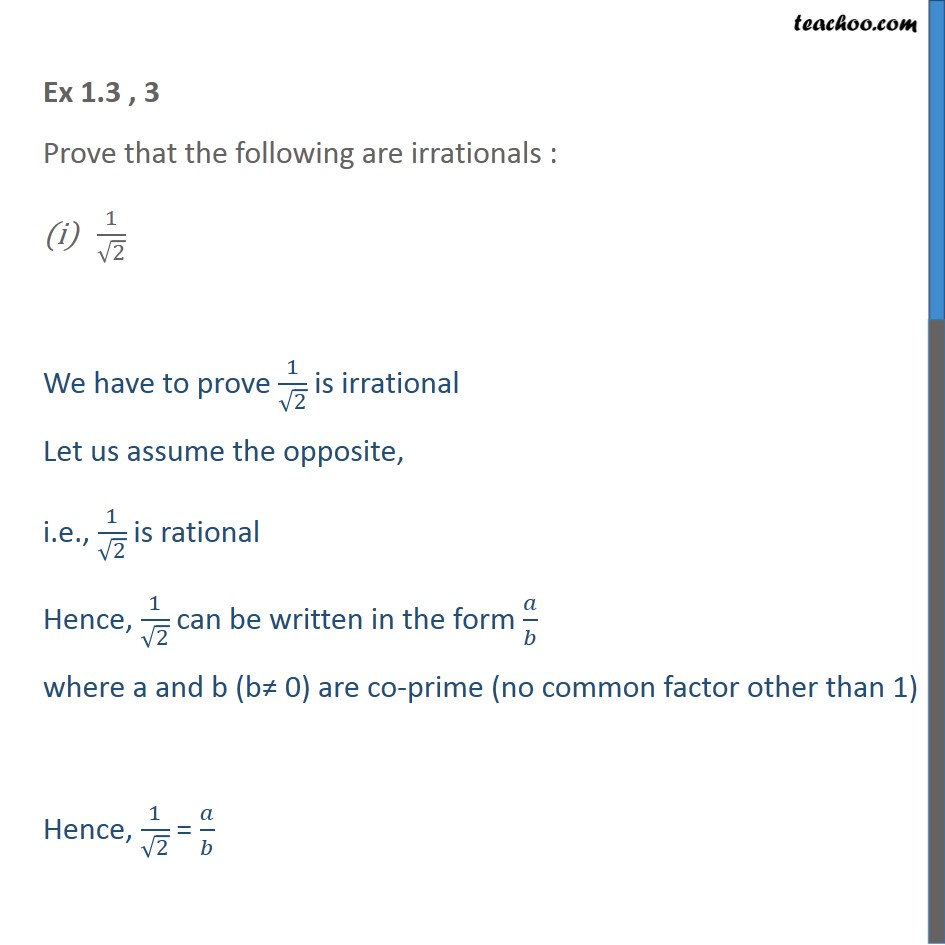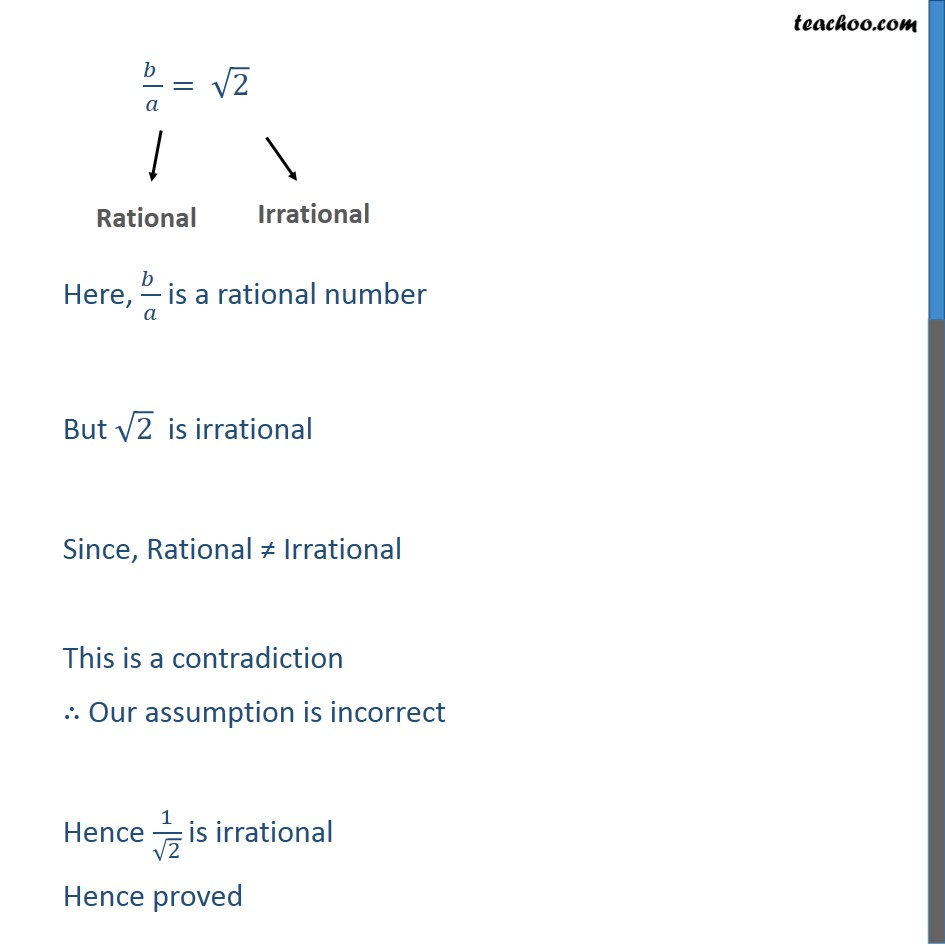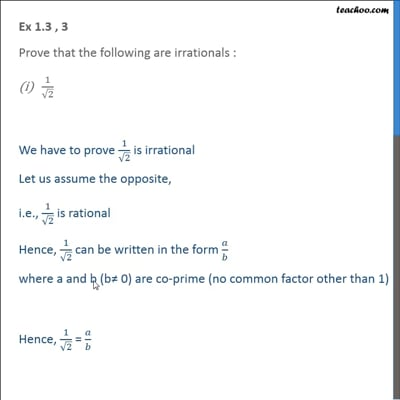Chapter 1 Class 10 Real Numbers

Class 10
Important Questions for Exam - Class 10This video is only available for Teachoo black users

Introducing your new favourite teacher - Teachoo Black, at only ₹83 per month

### Transcript

Ex 1.3 , 3 Prove that the following are irrationals : 1/√2 We have to prove 1/√2 is irrational Let us assume the opposite, i.e., 1/√2 is rational Hence, 1/√2 can be written in the form 𝑎/𝑏 where a and b (b≠ 0) are co-prime (no common factor other than 1) Hence, 1/√2 = 𝑎/𝑏 (𝑏 )/𝑎= √2 " " Here, (𝑏 )/𝑎 is a rational number But √2 is irrational Since, Rational ≠ Irrational This is a contradiction ∴ Our assumption is incorrect Hence 1/√2 is irrational Hence proved